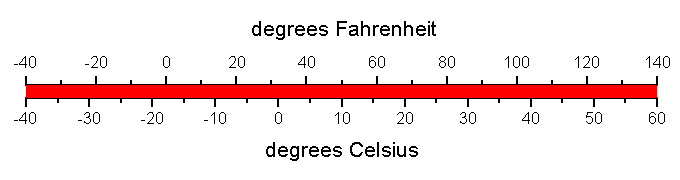Sidebar

# The Metric system

Every day of our lives, we talk about the size of things. "How much does that rock weigh? How tall is that building? How much water is in the container? How long will it take? How cold is it?" When we answer those questions, we use units. In the United States we typically use the English system. We measure distance in terms of inches, feet, and miles. We measure weight using ounces, pounds, and tons. Volumes are measured using ounces, quarts, and gallons. When we talk about temperature, we use degrees Fahrenheit. But the English system is not the only set of units; there is also the metric system.

The metric system is used by most countries and by all scientists. It is based on a decimal system and is designed to be simple. Let’s look at the metric units for four basic quantities: time, temperature, mass, and distance.

## Time - Seconds

The metric unit of time is the second, the same as the English system. That’s easy enough to remember. The second was originally defined as the 1/86,400 of a day. It’s about a heartbeat.

## Temperature - degrees Celsius

The metric temperature unit is degrees Celsius, instead of degrees Fahrenheit. The Celsius temperature scale is based on water. Zero degrees Celsius (C) is the freezing point of water and 100 degrees is the boiling point of water. The graph below compares the two temperature scales.There are simple equations to convert between Celsius and Fahrenheit:
Celsius = 5/9 x Fahrenheit – 32
Fahrenheit = 9/5 x Celsius + 32

## Distance - meters

The metric unit of distance is the meter. It is defined as the length of the path traveled by light in vacuum during a time interval of 1/299,792,458 of a second. Wow, that seems pretty complicated! Here's a simpler to think about the length of a meter: Take a big, giant step. That step was about a meter long. One meter is a little bit more than 3 feet. The graph below compares meters and feet.In the English system, distance is measured in inches, feet, yards, and miles. There are 12 inches in a foot, 3 feet in a yard, and 1760 yards in a mile. That's hard to remember and hard to compute. As we said earlier, the metric system is decimal based, and units are all divisible by ten. Check out the metric length examples.

Metric length examples

1 millimeter = 1/1000 meter = thickness of a fingernail
1 centimeter = 10 millimeters = 1/100 m = thickness of a finger
1000 millimeters = 100 centimeters = 1 meter = 1 giant step
1000 meters = 1 kilometer = 2 1/2 times around a track = 0.6 miles

## Mass - kilograms

The metric unit of mass is the kilogram. A kilogram is defined as the mass of one liter of water (a liter is the volume of a cube that's 0.1 m by 0.1 m by 0.1 m). A kilogram is equal to about 2.2 pounds. The prefix kilo means 1000, so kilogram means 1000 grams. A gram is pretty light, about the mass of a paperclip.

The metric system uses prefixes for different sizes of units. A few commonly used examples are

micro = 0.000001
milli = 0.001
centi = 0.01
kilo = 1000
mega = 1,000,000